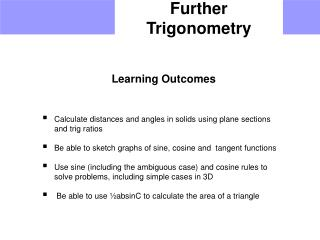DownloadDownload PresentationFurther Trigonometry

# Further Trigonometry

Télécharger la présentation## Further Trigonometry

- - - - - - - - - - - - - - - - - - - - - - - - - - - E N D - - - - - - - - - - - - - - - - - - - - - - - - - - -
##### Presentation Transcript

1. Further Trigonometry • Learning Outcomes • Calculate distances and angles in solids using plane sections and trig ratios • Be able to sketch graphs of sine, cosine and tangent functions • Use sine (including the ambiguous case) and cosine rules to solve problems, including simple cases in 3D • Be able to use ½absinC to calculate the area of a triangle

2. Further Trig Pythagoras’ Theorem & 3D Trig Consider the cuboid below • Find: • The length of the diagonal DG • The length of the diagonal FD • The angle the line FD makes with the base DCGH

3. x 4 7 Further Trig Revision of Trig & Pythagoras Find x on the following right angled triangles. 3. 1. 2. x 10 10 5 x 5 3 6. 4. 5. 4 7. x x 25º 30º 3.2 15 10 3 7.5 x x

4. B a c A b C Further Trig Sine Rule For a side For an angle

5. B 9 8 A b C Further Trig Sine Rule Find angle C. ? 50º

6. Further Trig Sine Rule Find the length of AC. A 62º 76º B ? 9 42º C

7. B a c A C b Further Trig Cosine Rule Use with non right angled triangles a2 = b2 + c2 – 2bc cos A

8. Further Trig Cosine Rule A 105º 8.1 5.2 x C B B 11.4 4.8 x A C 8.5